# 银行、金融

## 1、数据模型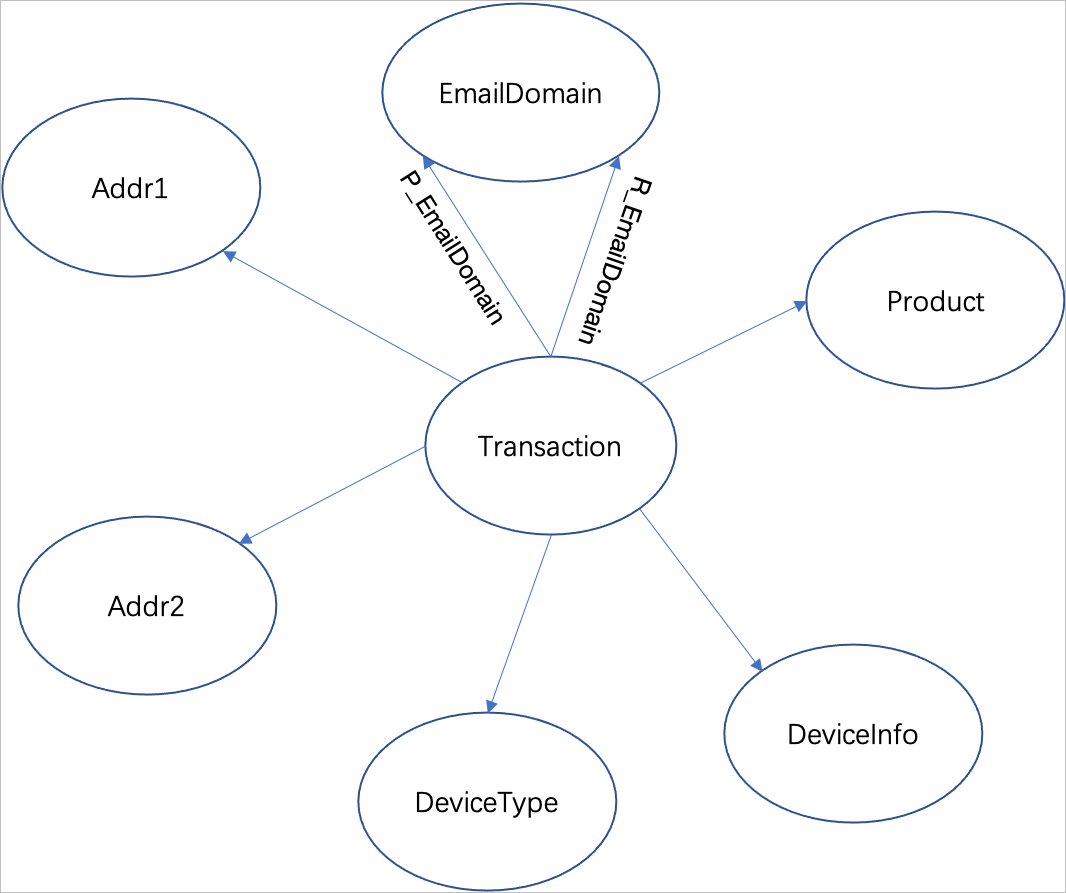• 点文件：

``````//Transaction交易信息。
~id,~label,is_fraud:int
2987000,"transaction",0
2987001,"transaction",0
2987002,"transaction",0
2987003,"transaction",0
2987004,"transaction",0

//DeviceType设备类型信息。
~id,~label
"mobile","devicetype"
"desktop","devicetype"``````
• 边文件：

``````~id,~from,~to,~label
1,2987000,"","t_e_p"
2,2987000,"","t_e_r"
3,2987000,"315.0","t_a1"
4,2987000,"87.0","t_a2"``````

## 2、创建实例

• 实例购买成功后，您可以在实例列表页面查看实例相关信息。通常，实例创建成功需要3~5分钟。

• 创建GDB实例后，您需要创建账号和密码、设置安全组，保证您具有GDB实例的访问权限。具体操作，请参见创建账号设置白名单

## 4、连接实例

• 通过GDB控制台直接登录数据管理服务DMS，更加方便快捷地远程访问、在线管理您的GDB数据库。具体操作，请参见通过DMS登录GDB数据库

• 通过开源组件GDB Console可视化控制台登录图数据库，可视化界面，操作简单，并可根据业务需求对可视化界面进行二次开发。具体操作，请参见通过开源组件GDB Console登录图数据库

• 通过Gremlin Console连接实例，命令行模式，适合Gremlin内核版本，适合用于查询语句性能优化。具体操作，请参见通过Gremlin Console连接实例

• 通过Cypher Shell连接实例，命令行模式，适合Cypher内核版本。具体操作，请参见通过Cypher Shell连接实例

• 通过SDK连接，支持Java、Python、.Net、Go、Node.js五种SDK。具体操作，请参见SDK参考

## 5、使用范例

• 简单查询

• 数据统计：

``````//统计点的数目。
gremlin> g.V().count()
==>592789

//统计边的数目。
gremlin> g.E().count()
==>2533038``````
• 过滤查询、排序查询：

``````//查询ID为2987000的交易信息。
gremlin> g.V('2987000').valueMap(true)
==>[id:2987000,label:transaction,is_fraud:]

//查询ID为2987000的交易信息。
gremlin> g.V('2987000').outE()
==>e[2987000-t_a1->315.0]
==>e[2987000-t_a2->87.0]
==>e[2987000-t_p->W]``````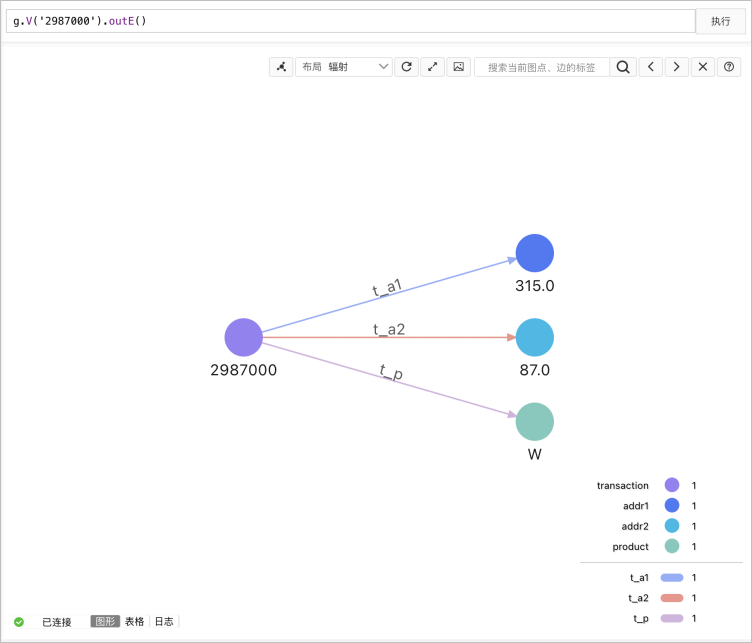• 通用场景

• K阶邻居：

``````//查询和交易记录2987000有相同地址的其他交易记录。
gremlin> g.V('2987000').repeat(bothE('t_a1').otherV().simplePath()).times(2).path() //bothE() 部分控制查询边类型，times()部分控制查询深度。
==>[v,e[2987000-t_a1->315.0],v[315.0],e[2987015-t_a1->315.0],v]
==>[v,e[2987000-t_a1->315.0],v[315.0],e[2987031-t_a1->315.0],v]
==>[v,e[2987000-t_a1->315.0],v[315.0],e[2987036-t_a1->315.0],v]
==>[v,e[2987000-t_a1->315.0],v[315.0],e[2987056-t_a1->315.0],v]
==>[v,e[2987000-t_a1->315.0],v[315.0],e[2987066-t_a1->315.0],v]
==>[v,e[2987000-t_a1->315.0],v[315.0],e[2987073-t_a1->315.0],v]
==>[v,e[2987000-t_a1->315.0],v[315.0],e[2987075-t_a1->315.0],v]
==>[v,e[2987000-t_a1->315.0],v[315.0],e[2987083-t_a1->315.0],v]
==>[v,e[2987000-t_a1->315.0],v[315.0],e[2987094-t_a1->315.0],v]
......``````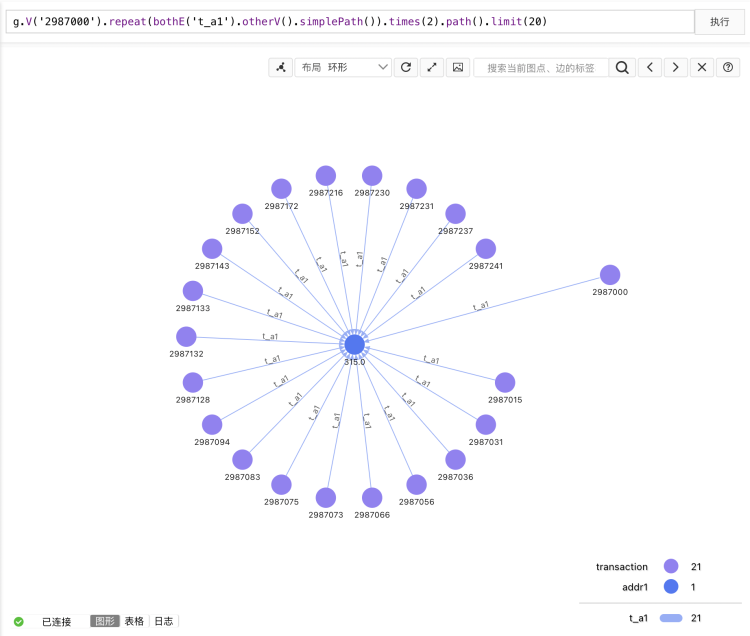• 最短路径：

``````//查询交易记录2987000和交易记录2987172的最短路径，最大深度为2。
gremlin> g.V('2987000').repeat(bothE().otherV().simplePath())
.until(hasId('2987172').or().loops().is(gt(2L)))  //hasId()部分控制结束ID，gt()部分查询深度。
.hasId('2987172').path().dedup()
==>[v,e[2987000-t_a1->315.0],v[315.0],e[2987172-t_a1->315.0],v]
==>[v,e[2987000-t_a2->87.0],v[87.0],e[2987172-t_a2->87.0],v]
==>[v,e[2987000-t_p->W],v[W],e[2987172-t_p->W],v]``````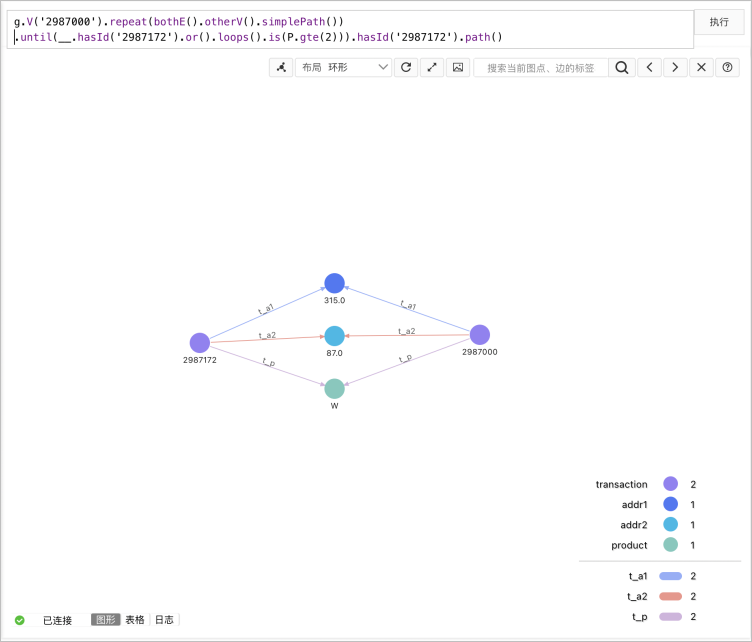• 共同邻居：

``````//查询交易记录2987000和交易记录2987172的共同邻居，从而找到具备相同属性（地址、设备）的交易记录。
gremlin> g.V('2987000').repeat(bothE().otherV().simplePath()).times(2).hasId('2987172').path().dedup() //hasId()部分控制结束ID。
==>[v,e[2987000-t_a1->315.0],v[315.0],e[2987172-t_a1->315.0],v]
==>[v,e[2987000-t_a2->87.0],v[87.0],e[2987172-t_a2->87.0],v]
==>[v,e[2987000-t_p->W],v[W],e[2987172-t_p->W],v]``````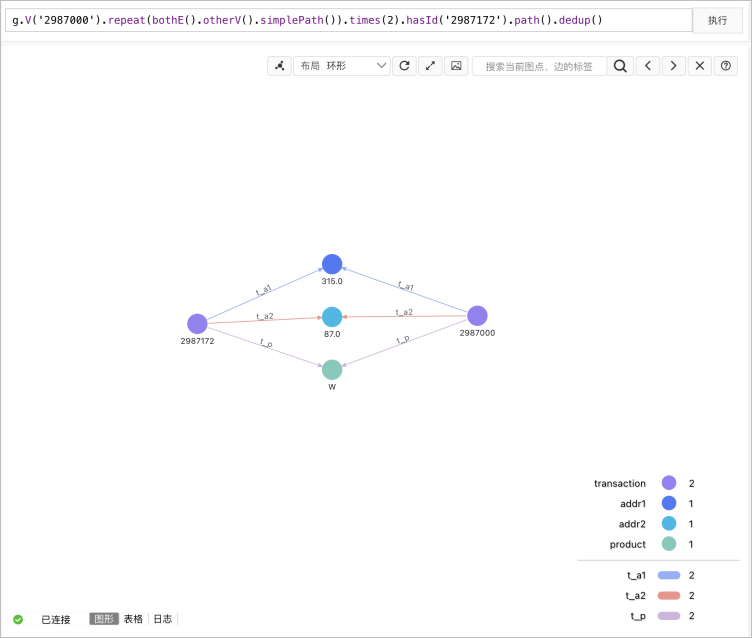• Jaccard相似度：

``````//查询和交易记录2987000相似的交易记录，根据相似度分数取前10。
gremlin> g.V('2987000')
.sideEffect(out().store('v1n'))
.as('v1')
.select('v1n').unfold().in().limit(100).simplePath().dedup().as('v2')
.project('i', 'u')
.by(select('v2').out().where(within('v1n')).count())
.by(union(select('v2').out().fold(),select('v1n')).unfold().dedup().count())
.project('Transaction', '相似度')
.by(select('v2').id())
.by(math('i/u'))
.order().by(select('相似度'), desc).limit(10)
==>[Transaction:2987015,相似度:1.0]
==>[Transaction:2987056,相似度:1.0]
==>[Transaction:2987216,相似度:1.0]
==>[Transaction:2987251,相似度:1.0]
==>[Transaction:2987371,相似度:1.0]
==>[Transaction:2987474,相似度:1.0]
==>[Transaction:2987699,相似度:1.0]
==>[Transaction:2987707,相似度:1.0]
==>[Transaction:2987735,相似度:1.0]
==>[Transaction:2987770,相似度:1.0]``````